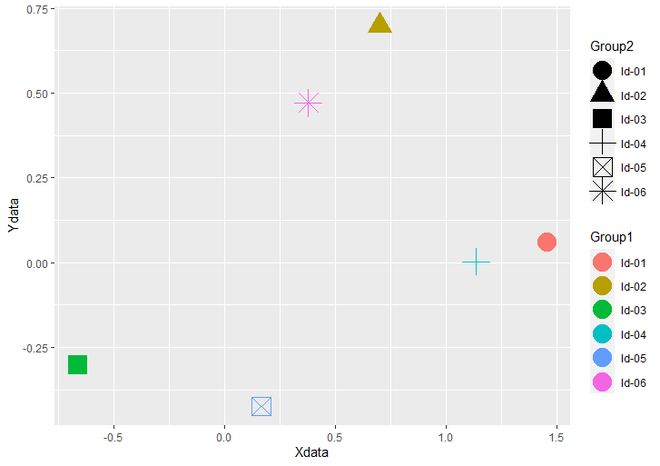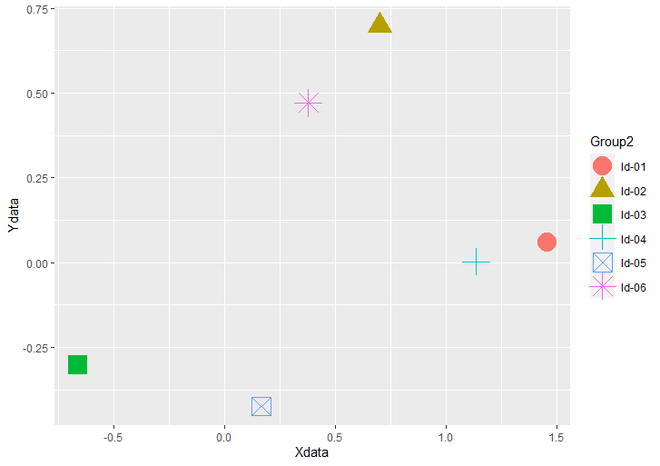# Combine Multiple ggplot2 Legend in R

• Last Updated : 23 May, 2021

In this article, we will see how to combine multiple ggplot2 Legends in the R programming language.

### Installation

First, load the ggplot2 package by using the library() function. If you have not installed it yet, you can simply install it by writing the below command in R Console.

`install.packages("ggplot2")`

To create an R plot, we use ggplot() function and for make it scatter plot we add geom_point() function to ggplot() function. Let us first create a plot with multiple legends in the same plot without combining so that the difference is apparent.

Example:

## R

 `# Load Package``library``(``"ggplot2"``)`` ` `# Create a DataFrame ``data <- ``data.frame``(Xdata = ``rnorm``(6),                        ``                   ``Ydata = ``rnorm``(6),``                   ``Group1 = ``c``(``"ld-01"``, ``"ld-02"``, ``"ld-03"``,``                              ``"ld-04"``, ``"ld-05"``, ``"ld-06"``),``                    ` `                   ``Group2 = ``c``(``"ld-01"``, ``"ld-02"``, ``"ld-03"``,``                              ``"ld-04"``, ``"ld-05"``, ``"ld-06"``))`` ` `# Create a Scatter Plot With Multiple Legends``ggplot``(data, ``aes``(Xdata, Ydata, color = Group1, shape = Group2)) +   ``  ``geom_point``(size = 7)`

Output:Scatterplot with multiple legends

As you can see in the above plot the two legends Group1 represents color and Group2 represents shape of Points in scatter plot are differently outlined. To Combine them into one legend, We should choose only one out of both Legends. Here we have chosen Group2, So we assign Group2 to color and shape parameters of aes() function. You can also choose Group1.

Example:

## R

 `# Load Package``library``(``"ggplot2"``)`` ` `# Create a DataFrame ``data <- ``data.frame``(Xdata = ``rnorm``(6),                        ``                   ``Ydata = ``rnorm``(6),``                   ``Group1 = ``c``(``"ld-01"``, ``"ld-02"``, ``"ld-03"``, ``                              ``"ld-04"``, ``"ld-05"``, ``"ld-06"``),``                    ` `                   ``Group2 = ``c``(``"ld-01"``, ``"ld-02"``, ``"ld-03"``,``                              ``"ld-04"``, ``"ld-05"``, ``"ld-06"``))`` ` `# Create a Scatter Plot with Combined``# multiple legends``ggplot``(data, ``aes``(Xdata, Ydata, color = Group2, shape = Group2)) +   ``  ``geom_point``(size = 7)`

Output:Scatteplot with Combined multiple Legends

My Personal Notes arrow_drop_up